Aug 18

# Elliptical Slice Sampling for priors with non-zero mean

I’ve been playing around with elliptical slice sampling (ESS) lately.  This is a new MCMC technique developed by Iain Murray, Ryan Adams, and David MacKay. Here is the original AISTATS paper. The punchline is that this method allows exact joint sampling for vectors whose posterior distribution can be expressed using any Gaussian prior and any (non-conjugate) likelihood. ESS does not have any free parameters, and always accepts its proposal.  This makes it favorable compared to, say, Metropolis-Hastings methods, since tuning the lengthscales that control a random walk to achieve good mixing rates requires expensive human time.

One tricky aspect of this method is that it requires a change of variables when applied to sample a variable with non-zero prior mean.  This is mentioned in the original paper, but not all that coherently. I thought this deserved a blog post, so that practitioners make sure they get it right.  The necessary steps are quite simple for experienced statisticians, so there’s nothing really novel or exciting happening. Read on for more.  Hopefully in the near future I’ll report on experiments comparing ESS to other methods on some more interesting models.

## Change of Variables

Suppose we wish to draw variable of interestfrom its posterior:, where the prior is Gaussian with meanand covariance, and the likelihoodis some generic function of.

Ifhas zero-mean prior, we can easily use ESS as a “black box” to get posterior draws, by iterating the following for many samples• For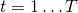•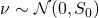•where the variabledefines the ellipse that makes ESS possible (see original paper for details).

However, ifhas a non-zero mean Gaussian prior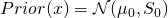, one might be tempted to modify the above procedure as follows:

• For•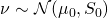(WRONG!)
•This is just flat out wrong, because ESS is *only* valid when we are sampling a variable that has a zero mean prior.

To overcome this obstacle, we simply change our variable of interestinto, which has zero mean, by simply setting.   However, we need redefine our likelihood function to compensate for this change, so that it “adds back in” this prior mean.  Thus, we set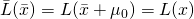.

Then, it is quite simple to get posterior drawsfromby repeating

• For••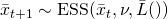After all samples are collected, set, so that the resulting samples are correctly centered.

## Toy Experiment

Here, I’ve completed a simple toy experiment to verify this procedure works as expected.  I’ve set up an easy problem, where both prior and likelihood forhave Gaussian distributions. This means we have a closed-form posterior for our variable, and thus we know the “right answer”.

I’ve then sampled many draws from this posterior, using the “CORRECT” change of variables method, and the “WRONG” method that naively adds the mean directly into theauxiliary variable within the ESS procedure.Clearly, the change of variables method correctly finds the mean of the posterior, while the WRONG “include the prior mean” method is way off.  So don’t be fooled, and make sure you do the change of variables correctly.

## Code to Try this Yourself

You can get a good matlab implementation of the basic ESS procedure from Iain Murray’s website: elliptical_slice.m

I then just used this matlab script to run this experiment and create the above plot:  ExploreESSWithMean.m

Along with this helper script for plotting the contours of Gaussian covariances: plotGauss.m